# JEE Mathematics Probability

• ### JEE Mathematics Probability MCQs Set D

Question: A and B throw a dice. The probability that the number that A's throw is not greater than B's throw is : a) 7/12 b) 1/2 c) 5/12 d) 1/6 Answer: 7/12 Question: From a pack of 52 cards two cards are drawn one by one atrandom, the probability that both the cards are aces is : a) 1/221...

• ### JEE Mathematics Online Test Probability Set F

Refer to JEE Mathematics Online Test Probability Set F below. Students of JEE Mathematics can refer to the full list of free JEE Mathematics Mock Test provided by StudiesToday. These MCQ based online mock tests for Chapter Probability in JEE Mathematics has been designed based on the pattern of...

• ### JEE Mathematics Online Test Probability Set E

Refer to JEE Mathematics Online Test Probability Set E below. Students of JEE Mathematics can refer to the full list of free JEE Mathematics Mock Test provided by StudiesToday. These MCQ based online mock tests for Chapter Probability in JEE Mathematics has been designed based on the pattern of...

• ### JEE Mathematics Online Test Probability Set D

Refer to JEE Mathematics Online Test Probability Set D below. Students of JEE Mathematics can refer to the full list of free JEE Mathematics Mock Test provided by StudiesToday. These MCQ based online mock tests for Chapter Probability in JEE Mathematics has been designed based on the pattern of...

• ### JEE Mathematics Online Test Probability Set C

Refer to JEE Mathematics Online Test Probability Set C below. Students of JEE Mathematics can refer to the full list of free JEE Mathematics Mock Test provided by StudiesToday. These MCQ based online mock tests for Chapter Probability in JEE Mathematics has been designed based on the pattern of...

# JEE Mathematics Probability MCQs Set D

#### Question: A and B throw a dice. The probability that the number that A's throw is not greater than B's throw is :

• a) 7/12
• b) 1/2
• c) 5/12
• d) 1/6

Click for more Probability Study Material
 JEE Mathematics Probability MCQs Set C JEE Mathematics Statistics and Probability MCQs JEE Mathematics Probability MCQs Set D JEE Mathematics Probability MCQs Set A JEE Mathematics Probability MCQs Set B

# JEE Mathematics Online Test Probability Set F

Refer to JEE Mathematics Online Test Probability Set F below. Students of JEE Mathematics can refer to the full list of free JEE Mathematics Mock Test provided by StudiesToday. These MCQ based online mock tests for Chapter Probability in JEE Mathematics has been designed based on the pattern of questions expected to come in the upcoming JEE Mathematics examinations. Students should also go through MCQs for JEE Mathematics Probability and also MCQ Questions for JEE Mathematics with answers for all other chapters.

 Questions: 14 Attempts allowed: Unlimited Available: Always Pass rate: 50 % Backwards navigation: Allowed
Start Online Test
 JEE Mathematics Online Test Probability Set A JEE Mathematics Online Test Probability Set B JEE Mathematics Online Test Probability Set C JEE Mathematics Online Test Probability Set D JEE Mathematics Online Test Probability Set E JEE Mathematics Online Test Probability Set F

# JEE Mathematics Online Test Probability Set E

Refer to JEE Mathematics Online Test Probability Set E below. Students of JEE Mathematics can refer to the full list of free JEE Mathematics Mock Test provided by StudiesToday. These MCQ based online mock tests for Chapter Probability in JEE Mathematics has been designed based on the pattern of questions expected to come in the upcoming JEE Mathematics examinations. Students should also go through MCQs for JEE Mathematics Probability and also MCQ Questions for JEE Mathematics with answers for all other chapters.

 Questions: 15 Attempts allowed: Unlimited Available: Always Pass rate: 50 % Backwards navigation: Allowed
Start Online Test
 JEE Mathematics Online Test Probability Set A JEE Mathematics Online Test Probability Set B JEE Mathematics Online Test Probability Set C JEE Mathematics Online Test Probability Set D JEE Mathematics Online Test Probability Set E JEE Mathematics Online Test Probability Set F

# JEE Mathematics Online Test Probability Set D

Refer to JEE Mathematics Online Test Probability Set D below. Students of JEE Mathematics can refer to the full list of free JEE Mathematics Mock Test provided by StudiesToday. These MCQ based online mock tests for Chapter Probability in JEE Mathematics has been designed based on the pattern of questions expected to come in the upcoming JEE Mathematics examinations. Students should also go through MCQs for JEE Mathematics Probability and also MCQ Questions for JEE Mathematics with answers for all other chapters.

 Questions: 15 Attempts allowed: Unlimited Available: Always Pass rate: 50 % Backwards navigation: Allowed
Start Online Test
 JEE Mathematics Online Test Probability Set A JEE Mathematics Online Test Probability Set B JEE Mathematics Online Test Probability Set C JEE Mathematics Online Test Probability Set D JEE Mathematics Online Test Probability Set E JEE Mathematics Online Test Probability Set F

# JEE Mathematics Online Test Probability Set C

Refer to JEE Mathematics Online Test Probability Set C below. Students of JEE Mathematics can refer to the full list of free JEE Mathematics Mock Test provided by StudiesToday. These MCQ based online mock tests for Chapter Probability in JEE Mathematics has been designed based on the pattern of questions expected to come in the upcoming JEE Mathematics examinations. Students should also go through MCQs for JEE Mathematics Probability and also MCQ Questions for JEE Mathematics with answers for all other chapters.

 Questions: 15 Attempts allowed: Unlimited Available: Always Pass rate: 50 % Backwards navigation: Allowed
Start Online Test
 JEE Mathematics Online Test Probability Set A JEE Mathematics Online Test Probability Set B JEE Mathematics Online Test Probability Set C JEE Mathematics Online Test Probability Set D JEE Mathematics Online Test Probability Set E JEE Mathematics Online Test Probability Set F

# JEE Mathematics Online Test Probability Set B

Refer to JEE Mathematics Online Test Probability Set B below. Students of JEE Mathematics can refer to the full list of free JEE Mathematics Mock Test provided by StudiesToday. These MCQ based online mock tests for Chapter Probability in JEE Mathematics has been designed based on the pattern of questions expected to come in the upcoming JEE Mathematics examinations. Students should also go through MCQs for JEE Mathematics Probability and also MCQ Questions for JEE Mathematics with answers for all other chapters.

 Questions: 14 Attempts allowed: Unlimited Available: Always Pass rate: 50 % Backwards navigation: Allowed
Start Online Test
 JEE Mathematics Online Test Probability Set A JEE Mathematics Online Test Probability Set B JEE Mathematics Online Test Probability Set C JEE Mathematics Online Test Probability Set D JEE Mathematics Online Test Probability Set E JEE Mathematics Online Test Probability Set F

# JEE Mathematics Online Test Probability Set A

Refer to JEE Mathematics Online Test Probability Set A below. Students of JEE Mathematics can refer to the full list of free JEE Mathematics Mock Test provided by StudiesToday. These MCQ based online mock tests for Chapter Probability in JEE Mathematics has been designed based on the pattern of questions expected to come in the upcoming JEE Mathematics examinations. Students should also go through MCQs for JEE Mathematics Probability and also MCQ Questions for JEE Mathematics with answers for all other chapters.

 Questions: 15 Attempts allowed: Unlimited Available: Always Pass rate: 50 % Backwards navigation: Allowed
Start Online Test
 JEE Mathematics Online Test Probability Set A JEE Mathematics Online Test Probability Set B JEE Mathematics Online Test Probability Set C JEE Mathematics Online Test Probability Set D JEE Mathematics Online Test Probability Set E JEE Mathematics Online Test Probability Set F

# JEE Mathematics Statistics and Probability MCQs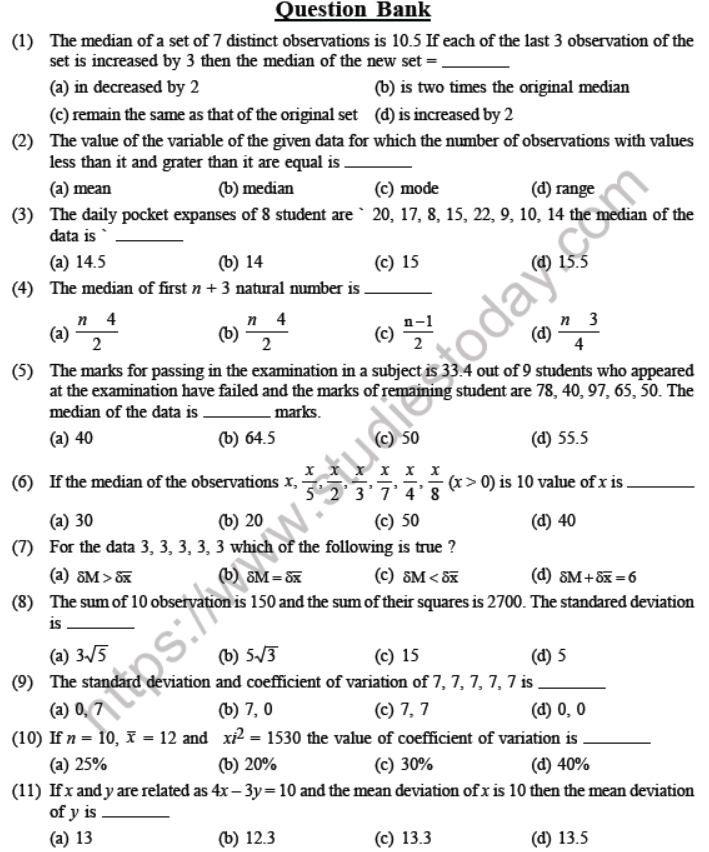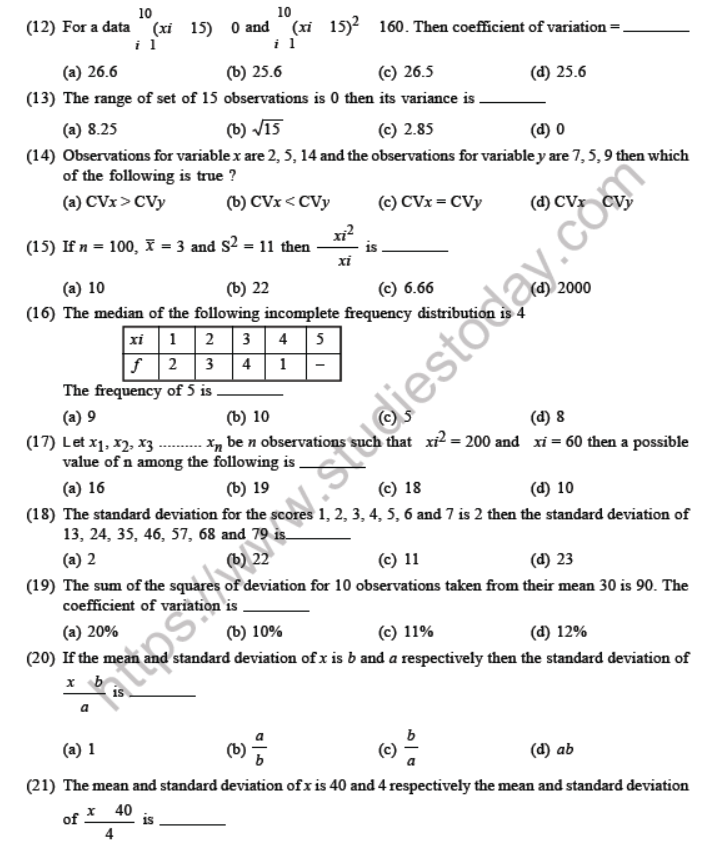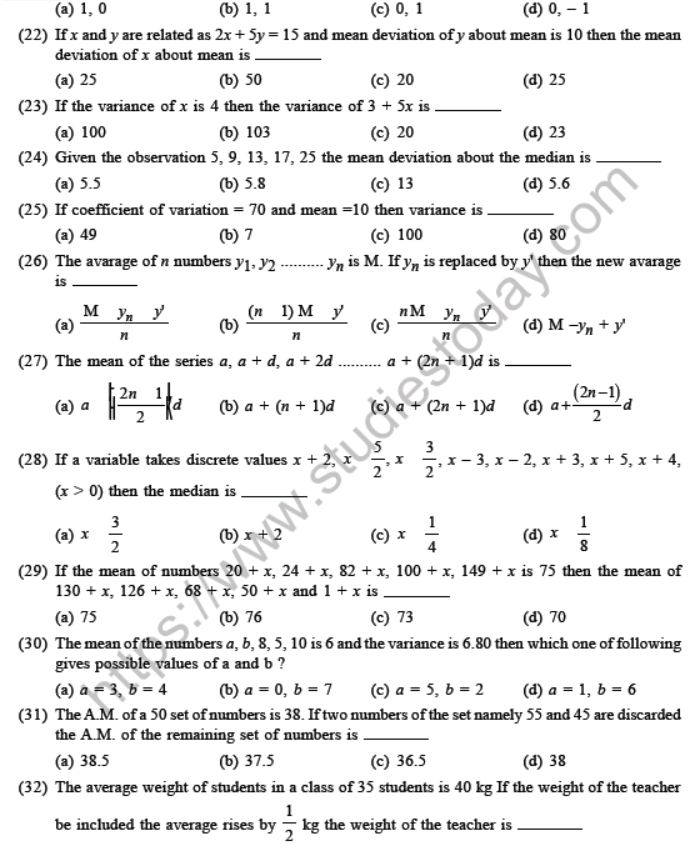Click for more Probability Study Material
 JEE Mathematics Probability MCQs Set C JEE Mathematics Statistics and Probability MCQs JEE Mathematics Probability MCQs Set D JEE Mathematics Probability MCQs Set A JEE Mathematics Probability MCQs Set B

# JEE Mathematics Probability MCQs Set C

Click for more Probability Study Material
 JEE Mathematics Probability MCQs Set C JEE Mathematics Statistics and Probability MCQs JEE Mathematics Probability MCQs Set D JEE Mathematics Probability MCQs Set A JEE Mathematics Probability MCQs Set B

# JEE Mathematics Probability MCQs Set B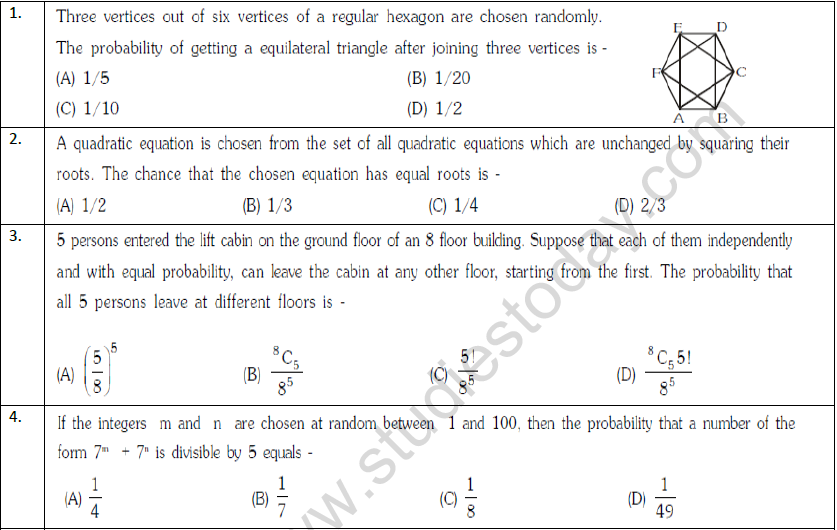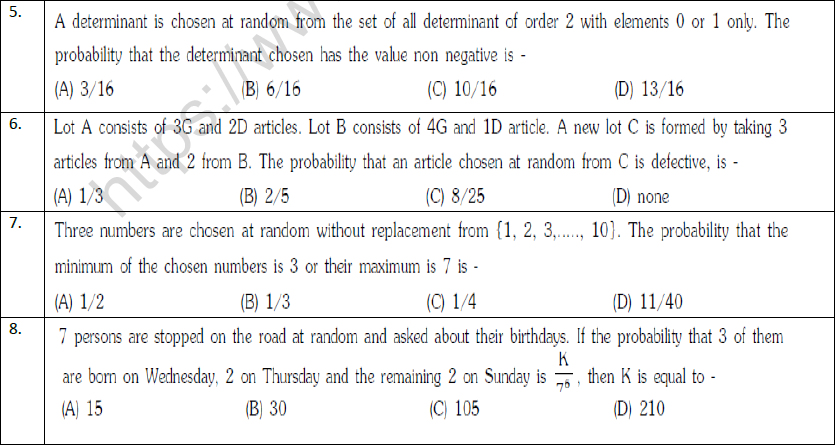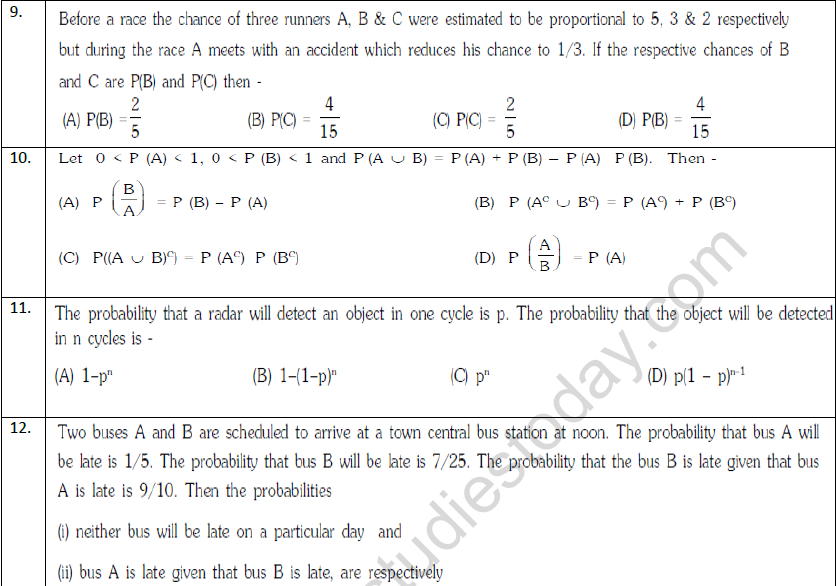Click for more Probability Study Material
 JEE Mathematics Probability MCQs Set C JEE Mathematics Statistics and Probability MCQs JEE Mathematics Probability MCQs Set D JEE Mathematics Probability MCQs Set A JEE Mathematics Probability MCQs Set B

## Latest NCERT & CBSE News

Read the latest news and announcements from NCERT and CBSE below. Important updates relating to your studies which will help you to keep yourself updated with latest happenings in school level education. Keep yourself updated with all latest news and also read articles from teachers which will help you to improve your studies, increase motivation level and promote faster learning

### CBSE Class 10 Revised Syllabus

Last year CBSE had to reduce the syllabus because of the pandemic situation but it was not very effective because there were no examinations. This year to avoid any confusion and conflict, CBSE has decided to reduce the syllabus into term 1 and term 2. 50 percent of...